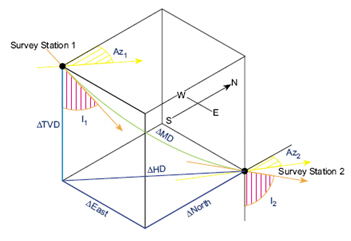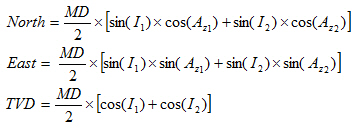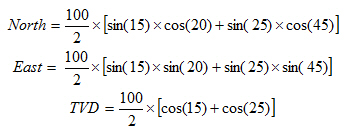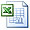# Balanced Tangential Method Calculation

I have some directional calculations that I’ve shared with you. However, some of them are not shared yet so I will try to share all directional drilling formulas that may be helpful for you in the future.

Today, I would like to show you about Balanced Tangential Method Calculation. This calculation method treats half of the measured distance (MD/2) as being tangent to I1 and Az1 and the remainder of the measured distance (MD/2) as being tangent to I2 and Az2.The formulas for the balanced tangential method are listed below;Where;

MD = Measured Depth between surveys in ft

I1 = Inclination (angle) of upper survey in degrees

I2 = Inclination (angle) of lower in degrees

Az1= Azimuth direction of upper survey

Az2 = Azimuth direction of lower survey

The following example is the Balanced Tangential Method Calculation.

Survey 1

Depth = 3500 ft

Inclination = 15 degree (I1)

Azimuth = 20degree (Az1)

Survey 2

Depth = 3600 ft

Inclination = 25 degree (I2)

Azimuth = 45 degree (Az2)

Solution:

MD = 3600 – 3500 = 100 ft

As per the formulas above, you will get answers as listed below;North = 27.1 ft

East = 19.37 ft

TVD = 93.61 ft

Please find the Excel sheet used for TheBalanced Tangential Method Calculation

Share the joy